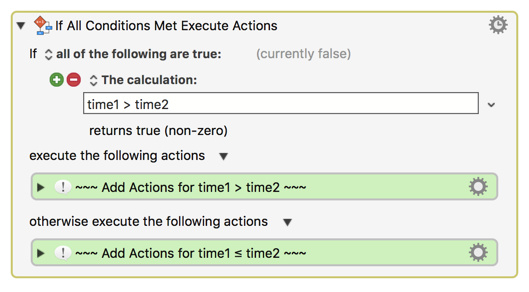# Compare two time values possible in KM?

Is it possible to compare two video time values in the form of hh:mm:ss:ff (i.e., hours:mins:secs:frames)?

I'm trying to automate something in After Effects so that if one give duration is less then 10 seconds long then have KM perform a certain action.

Thanks!

Hey @project_guru,

You can't compare hh:mm:ss:ff except for string equality, but if you break it up into pieces you have more options.

See Calculations on the wiki.

-Chris

You will need to convert each video time value into a numeric total seconds, and then make the comparison using a KM If Then Else action, something like this:

``````set time1 to [convert video time 1 to total seconds]
set time2 to [convert video time 2 to total seconds]
``````To convert video time to total seconds, see:
MACRO: Add or Subtract SMPTE TimeCode Strings [Example]

This macro contains a script that does the conversion from SMPTE time to total seconds (or total frames).

Questions?